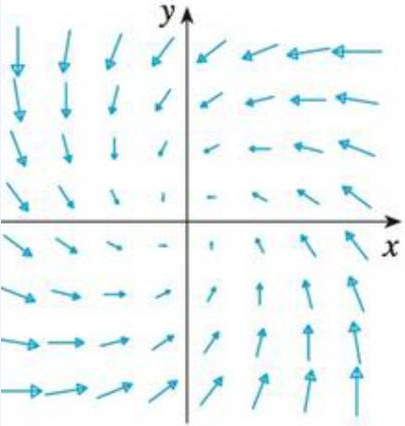Chapter 16.3, Problem 25E

Chapter
Section
Textbook Problem

Is the vector field shown in the figure conservative? Explain.To determine

To explain: Whether the vector field shown in the figure is conservative or not.

Explanation

Definition:

For a conservative vector field (F) , the line integral around any closed path C is zero. The conservative vector field can be mathematically expressed as follows:

CFdr

Still sussing out bartleby?

Check out a sample textbook solution.

See a sample solution

The Solution to Your Study Problems

Bartleby provides explanations to thousands of textbook problems written by our experts, many with advanced degrees!

Get Started

Evaluate the indefinite integral. sec22d

Single Variable Calculus: Early Transcendentals, Volume I

Evaluate the integral. tan5xdx

Calculus (MindTap Course List)

True or False: If f(x) = f (−x) for all x then .

Study Guide for Stewart's Single Variable Calculus: Early Transcendentals, 8th

Find the sum of first 20 terms of the arithmetic progression 5,1,7,

Finite Mathematics for the Managerial, Life, and Social Sciences